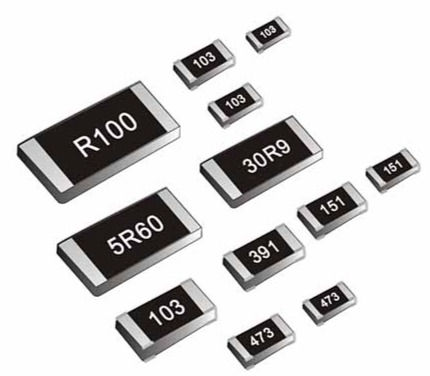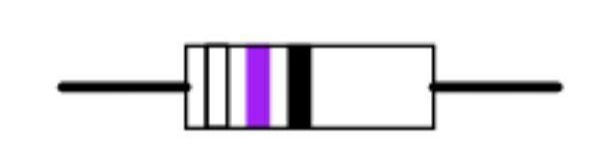top of page

# Resistors & Its Resistance

Updated: Jul 15, 2021

All of us know that when the #electric current is passed through any device or conductor, heat will be generated. But have you ever wondered how the appliances are working continuously without any damage even upon passing high voltage? The reason is nothing but the presence of resistors in the circuit. In general, the resistors are capable of controlling the flow of electric #current thereby preventing the damage of the device.

We can construct #resistors with specific values according to our requirement and at present, there are millions of resistors available in the market. In this article, we are going to discuss in detail the resistors, the unit of resistors, and the way in which values are assigned to it, its applications.

### Resistors

Technically speaking, resistors are passive two-terminal electrical components that implement electrical #resistance as their circuit element. Apart from reducing the current flow, they also perform functions like adjusting signal levels, terminate transmission lines, dividing voltages, and biasing active elements. Resistors are common elements of electric circuits, electrical networks and are also implemented within integrated circuits.Resistors are passive components (i.e) they are only capable of consuming power but can't generate it. Resistors can be constructed out of a variety of elements which include carbon, metal, or any metal-oxide film compositions.

Mostly the resistors are connected either in series or in the parallel combination.

In series combination, the total resistance of the resistors is merely the sum of their individual resistances.

Whereas in parallel combination, the total resistance of the resistors connected is the reciprocal of the sum of the reciprocals of the individual resistors.

A resistor network that is a combination of both series and parallel connections can be solved using the matrix method or Y-Δ transform.

### Relation of resistors with ohm's law

The #ohm (symbol: Ω) is the unit of electrical resistance, named after Georg Simon Ohm. An ohm is equivalent to a volt per ampere. Practical resistors also own a certain amount of capacitance and inductance which affect the relation between voltage and current in AC circuits. The behavior of an ideal resistor can be explained in detail with the help of ohm's law.

The law states that the voltage (v) across a resistor is proportional to the current (I), while the constant of proportionality is resistance (R).

By using ohm's law, one can easily find how much amount of voltage can be passed through a resistor such that:

## V=I.R

In this modern era of #electronics, resistors are manufactured over a very large range of values and so the derived units such as milliohm, megaohm, and kiloohm are now very common in usage.

### Types of resistors

Based on the size and shape, the resistors are classified into two types. They are PTH (Plated Through Hole) and SMD (Surface Mount Device). Through-hole resistors are the ones with long, pliable leads that can be stuck into a breadboard or any printed #circuit boards.

These kinds of resistors are normally used in experiments performed in educational institutions and also in small electric toys, in remotes used for controlling the television, air conditioners.SMD resistors are usually tiny black rectangular-shaped resistors with shiny silver conductive edges. They are intended to sit on the top of PCBs usually placed by robots and sent through the oven. The solder melts and holds them in place. The SMD resistors are employed in commercial electronics such as in circuits of refrigerators, washing machines, and also in several industrial machines.Further, the resistors are classified as fixed resistors and variable resistors based on the value of resistance. The value of fixed resistors is determined during the design phase of the circuit and it cannot be changed again till the lifespan of the circuit is over.

While the variable resistors consist of a fixed resistor element and a slider that taps onto the main resistor element. Though this type of configuration, it can provide variable resistance to the resistor depending on the flow of current.

Other than the above-mentioned types, there are also unique resistors classified based on their specialized applications. Light-dependent or photoresistors which can change its resistance value depending on the intensity of light. Thermisters whose resistance constantly varies depending on the temperature. Varistor whose resistance changes depending on the applied voltage. wire wound resistors, carbon composition resistors are also some kind of resistors.

### Standard Color Codes

Resistor color-coding uses colored bands to identify the resistance value of resistors quickly along with the percentage of tolerance. The following table explains the value and tolerance levels of respective colors. 4-band and 5-band code resistors are the two kinds of resistors that are used almost.For 4-band resistors, gold, silver, and none are only three identities used for indicating the tolerance level. Whereas in 5-band resistors red, green, violet, brown, blue is used to indicate the tolerance level. For brown color, the tolerance level is 1%, red 2%, green 0.5%, blue 0.25%, violet 0.1%, gold 5%, silver 10%, no indication of color 20%. Let's discuss some examples to understand the concept of calculating the value of resistance using color codes.

Sample 1:The color code of this resistor is Green-Red-Gold-Silver. By multiplying the respective values given in the above table, the resistance of this resistor would be 5.2 ohms with a tolerance of about +/- 10%.

Sample 2:The color code of this resistor is White-Violet-Black. The resistance would be 97 ohms with a tolerance level of about +/- 20%. If there are only three color bands on a resistor, we should remind that it is actually a 4-band code with a blank (20%), tolerance band.

In our day-to-day life, almost all the electronic boards, and devices make use of the resistors. Appliances such as Mobile phones, laptop boards, juicer contains SMD resistors in them. CFL and other lighting lamps consist of metal film resistors. Further, the mobile phone and laptop charges also have resistors in them in order to control the flow of excessive current.